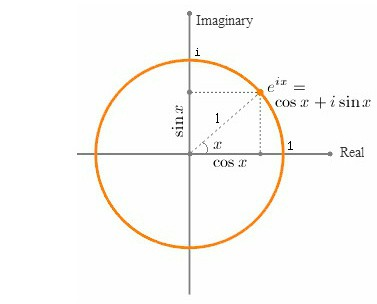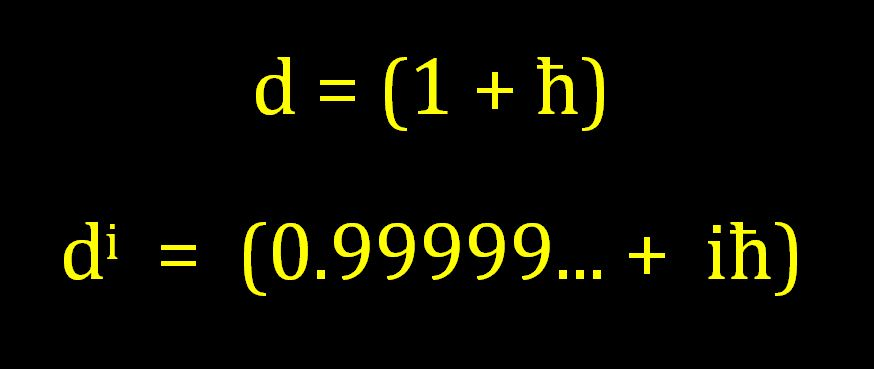# How Do You Like Them Apples?Named after the Swiss mathematician Leonhard Euler – Euler’s Identity is often described as an example of deep mathematical beauty.  Some see it almost as proof of the existence of some hidden order of the universe that we can only catch a glimpse of…

This equation is considered amazing because it seems to sets up a relationship between three apparently completely unrelated subjects.

“e” originated from the study of compound interest (and was subsequently found to be the base of the natural logarithms); “π” originated from Geometry and Trigonometry; and i” was “invented” to help with solving equations involving negative numbers.

Compound Interest, Trigonometry, and Imaginary Numbers?…  The question that has intrigued mathematicians and physicists since Euler first published his famous formula in 1748, is “Why in God’s name do these (apparently completely unrelated) areas of mathematics fit together so neatly in this beautiful equation?”

###### Well here’s the answer – Watch!

[Note: What follows is just a quick jump-thru, if you want the in-depth understanding click here]

e  =  Limit as n →  (1 + 1/n)n  =  2.718281828459045……

For practical purposes, “e” is difficult to pin down is simply a consequence of the fact that “e” is a manufactured quantity, defined using “limits to infinity”, and infinity is an idea not a number.

In reality there is no definitive value of “Transcendental” “e” because we can theoretically infinitely add more and more decimal places to the value of “e” by constantly increasing n all the way to infinite (which doesn’t actually exist).

However although the concept of infinity might be vague, “the concept of “e””, on the other hand, is actually all about very finely-grained precision…

The concept and formula for “e” is neat and very precise because the number of steps (n) must be the exact inverse of the tiny step-size (1/n).

In order to discuss the preciseness of “e”, it is better, for the purposes of this discussion, to limit the influence of “infinity” and define “e” with very precise numbers.

To do so, we will use both a very large number and a very small number which are exact inverses of each other.  We will use the symbol Ħ for the very large number, and we will use the symbol ħ for the very small number.

Ħ  =  10,000,000,000,000,000,000,000,000,000,000,000  =  10+34

ħ  =  0.000,000,000,000,000,000,000,000,000,000,000,1  =  10-34

Since ħ = 1/Ħ we can now write a precision equation for “e” as

e = ( 1  +  ħ )Ħ  =  2.718281828459045……

Okay, so now away we go…

e = ( 1  +  ħ )Ħ

e1/Ħ = ( 1  +  ħ )

eħ = ( 1  +  ħ )

======

Set       d  =  eħ  =  ( 1  +  ħ )

======

dxĦ  =  (1 + ħ)xĦ  =  (eħ)xĦ  =  (eħĦ)x  ex

so

d  =  (1 + ħ)1Ħ  (eħ)1Ħ  =  (eħĦ)1  =  e1  =  2.718281828459…

[Ħ micro-steps of (1+ħ) is equivalent to 1 macro-step of 2.71828]

Okay that was for real numbers, now let’s do complex numbers…

(d)i  =  (ex)i  =  cos(x) +  i sin(x)

(d)i  =  ei  =  cos(1) +  i sin(1)

di  =  eħi  =  cos(ħ) +  i sin(ħ)

di  =  (0.99999999… +  iħ)di(xĦ)   =   ( 1 + iħ )xĦ   =   (eiħ)xĦ   =   (eħĦ)ix   =   eix

so

di(1Ħ)   =   ( 1 + iħ )1Ħ   =   (eiħ)1Ħ   =   (eħĦ)i1   =   ei1

[Ħ micro-steps of (1+iħ) is equivalent to 1 macro-step of 1 Radian]

and

di(3.14159…Ħ)  =   ( 1 + iħ )πĦ   =   (eiħ)πĦ   =   (eħĦ)iπ   =   eiπ

[3.14159…Ħ micro-steps of (1+iħ) is equivalent to 1 macro-step of π Radians]

.

di(πĦ)    =    -1.00 + i0.00

⇒     di(πĦ)  =    -1

.

.

How do you like them apples?

So why is any of this important?

It is important because despite what is generally believed, Euler Identity is not telling us something special about “rotations”; it is telling us something special about “oscillations”.

[Remember: this was just a quick jump-thru; for a more detailed explanation click here.]

Euler’s identity is a beautiful example of the “quantized mathematics of change”.This quantization of mathematics allows us to define very precise “coarse” macro step-sizes (both linear and angular) using integer amounts of the quantized micro units d and di.

• d(xĦ) : defines the coarseness of linear growth and decay
• di(xĦ) : defines the coarseness of oscillations[This post is adapted from What is Euler’s Identity?.]## Author: Kieran D. Kelly

Experimental and Applied Mathematician, Specialist in Nonlinear Systems and Dynamics…